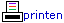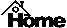Actueel
Archief
Culinair
Didactiek
Documentatie
Etalage
Formules
Functies
Geschiedenis
ICT
ICTauteur
Laatste nieuws
Lesmateriaal
Muziek
Natuur
Onderwijs
Ontspanning
Persoonlijk
Probleemaanpak
Proeftuin
Puzzels
Rekenen
Rekenmachines
Ruimtemeetkunde
Schoolwiskunde
Snippers
Systeem
Taal van de wiskunde
Vergelijkingen
Verhalen
WisFaq
WisKast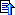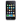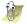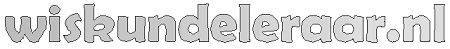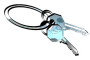## Omar Khayyįm's geometric solution of a cubic equation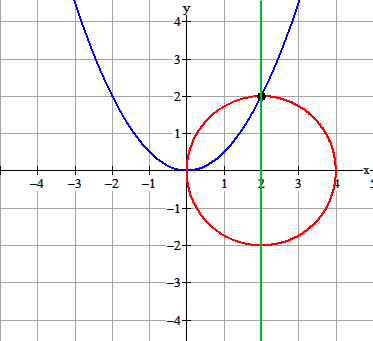"Omar Khayyám's geometric solution of a cubic equation, for the case $m = 2$, $n = 16$, giving the root $2$. The intersection of the vertical line on the $x$-axis at the center of the circle is happenstance of the example illustrated."

Voorbeeld

De vergelijking $x^3+4x=16$. Volgens Derive is de oplossing gelijk aan $x=2$. We zien $a=2$ en $b=16$.

Volgens Khayyam:

• Teken de parabool $y=\frac{1}{2}x^2$
• Teken de cirkel met een diameter van 4. Dat is $(x-2)^2+y^2=4$.
• Trek een verticale lijn door het snijpunt van de parabool en de cirkel.
• Lees de oplossing af.$\begin{array}{l} \left\{ \begin{array}{l} y = \frac{1}{2}x^2 \\ \left( {x - 2} \right)^2 + y^2 = 4 \\ \end{array} \right. \\ \left( {x - 2} \right)^2 + \left( {\frac{1}{2}x^2 } \right)^2 = 4 \\ x^2 - 4x + 4 + \frac{1}{4}x^4 = 4 \\ \frac{1}{4}x^4 + x^2 - 4x = 0 \\ x^4 + 4x^2 - 16x = 0 \\ x(x^3 + 4x - 16) = 0 \\ x = 0 \vee x^3 + 4x - 16 = 0 \\ x = 0 \vee x^3 + 4x = 16 \\ \end{array}$

 Opdracht Benader de oplossing van $x^3+16x=64$ m.b.v. de methode van Khayyam.